## How to Factor Quadratic Equations: Algebra

In this post, we are going to dive deep into how to factor Quadratic equations! There are so many different methods to choose from including GCF, Product/Sum, DOTS, and the Quadratic Formula.  Here we will go step by step into each method on how to factor quadratic equations, each with their own set of practice questions. For a review on how to factor by grouping, check out this post here and  happy calculating! 🙂

Why factor in the first place, you may say? We want to manipulate the equation until we solve for x.  Solving for x is our main goal, and factoring allows us to do that.  Now let’s get to the good stuff!## Greatest Common Factor (GCF):

The greatest common factor is the highest possible number that can be divided out from an equation.  This gets the equation into its simplest form and makes it easier for us to solve for x.

Before considering which type of factoring methdo to use, always ask yourself, “Can I take out a GCF?”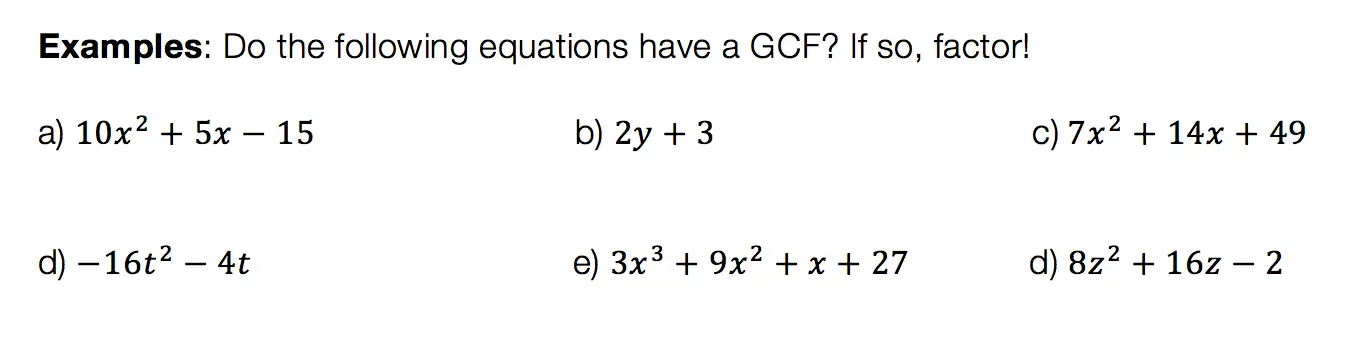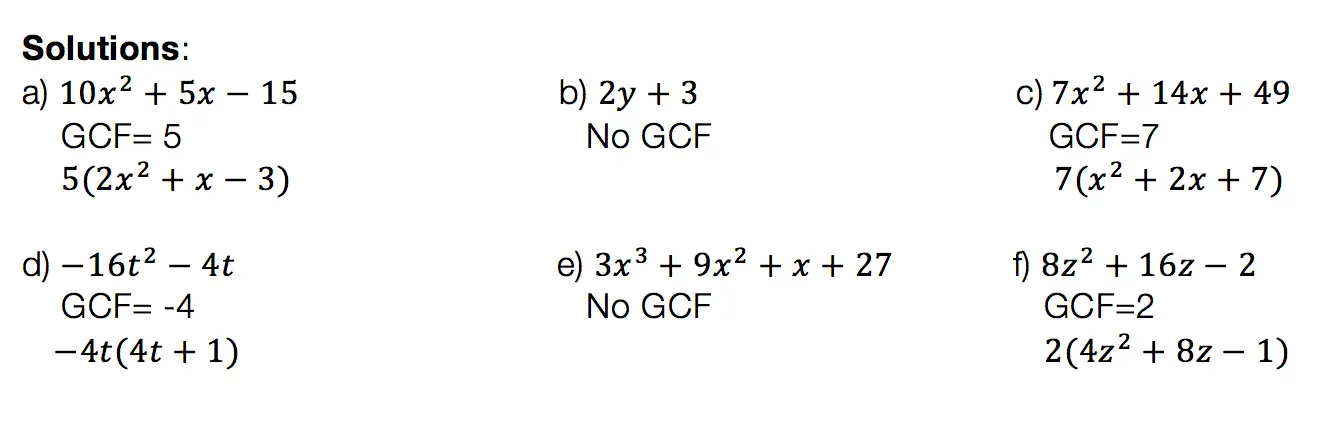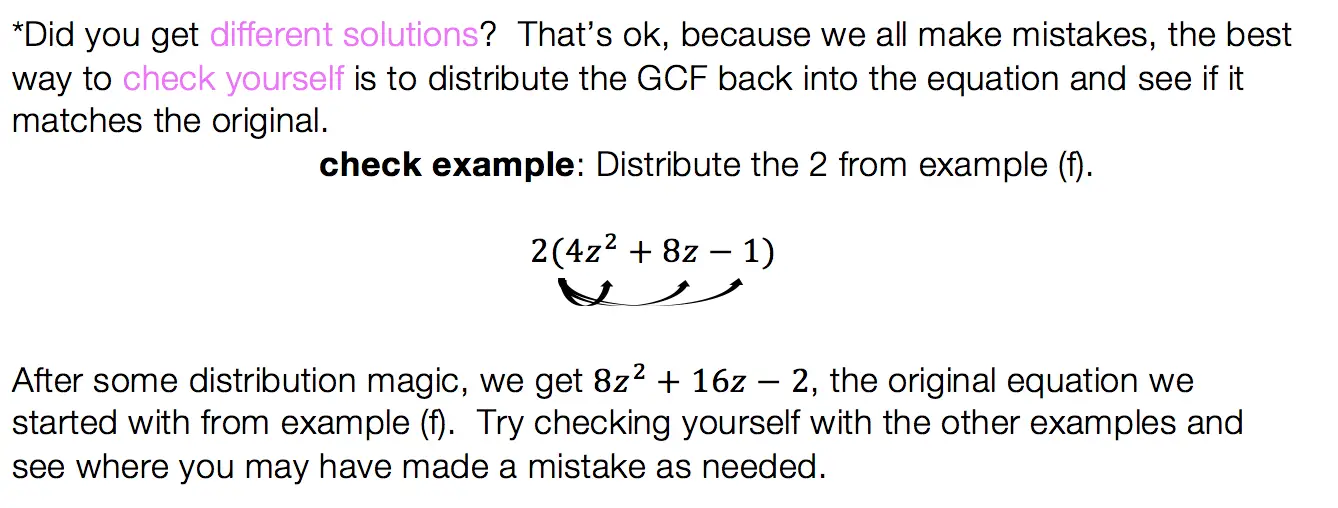## Product/Sum:

This factoring method is for quadratic equations only! That means the equation takes on the following form: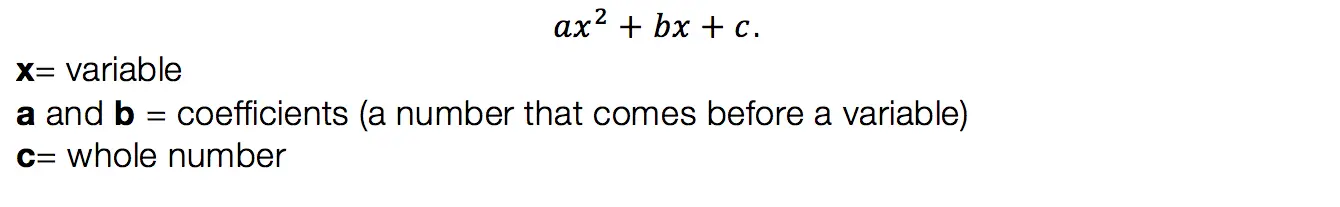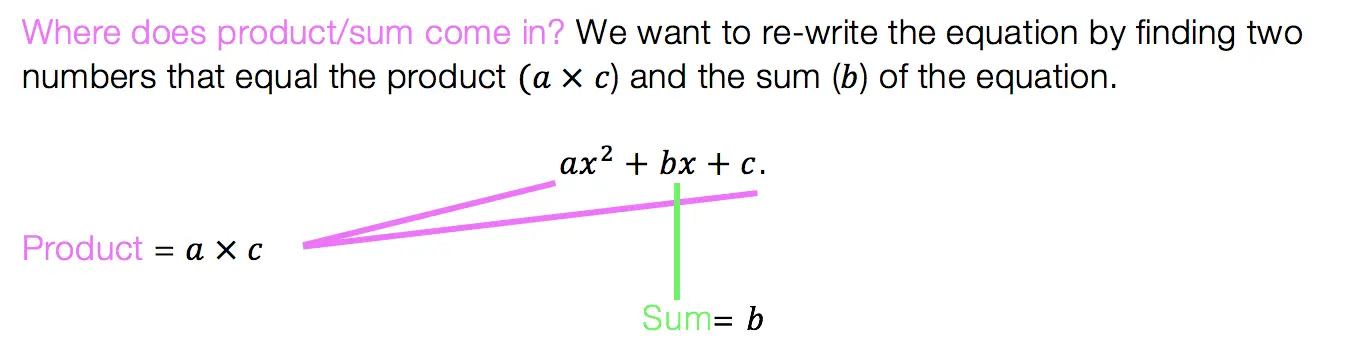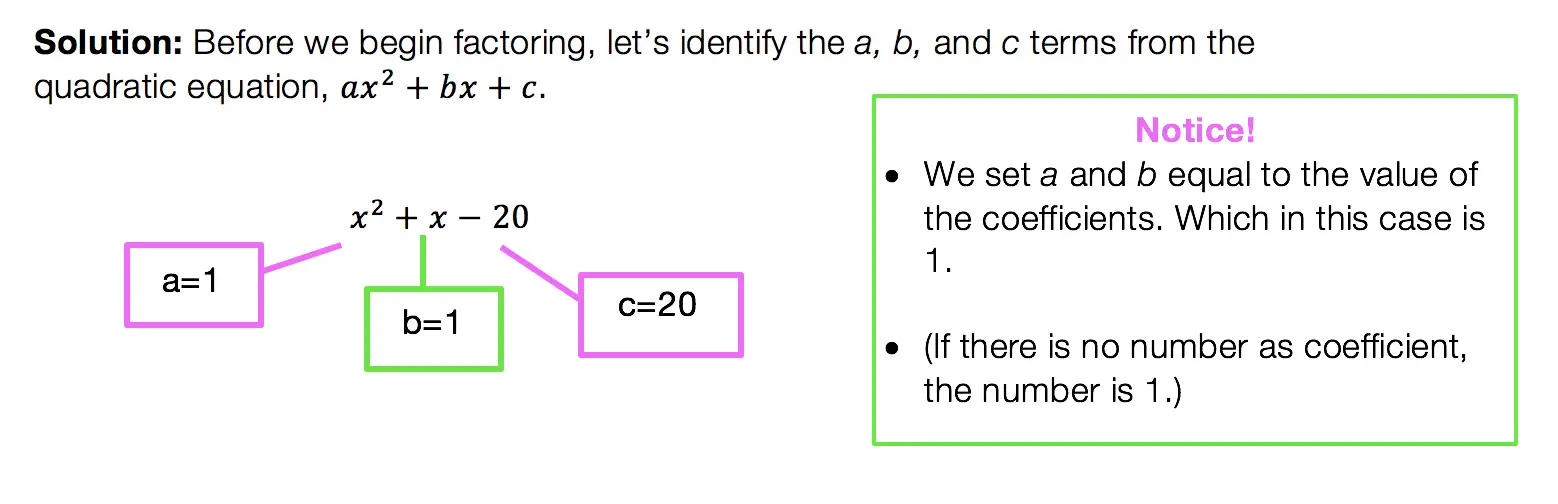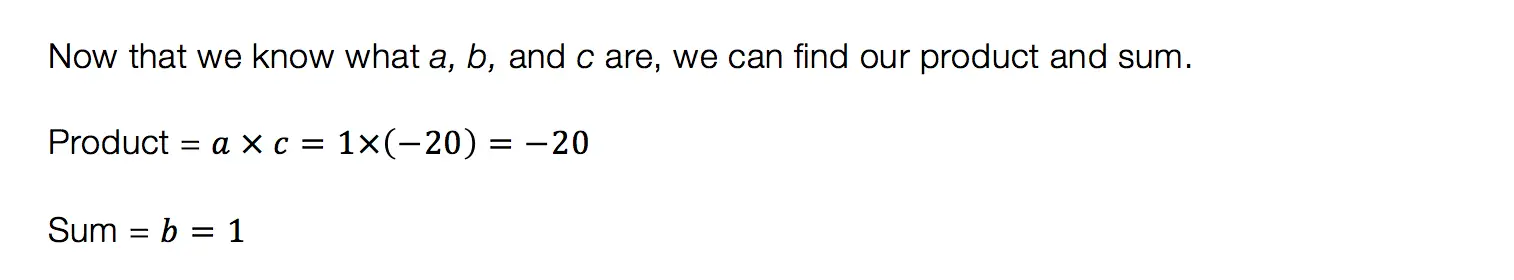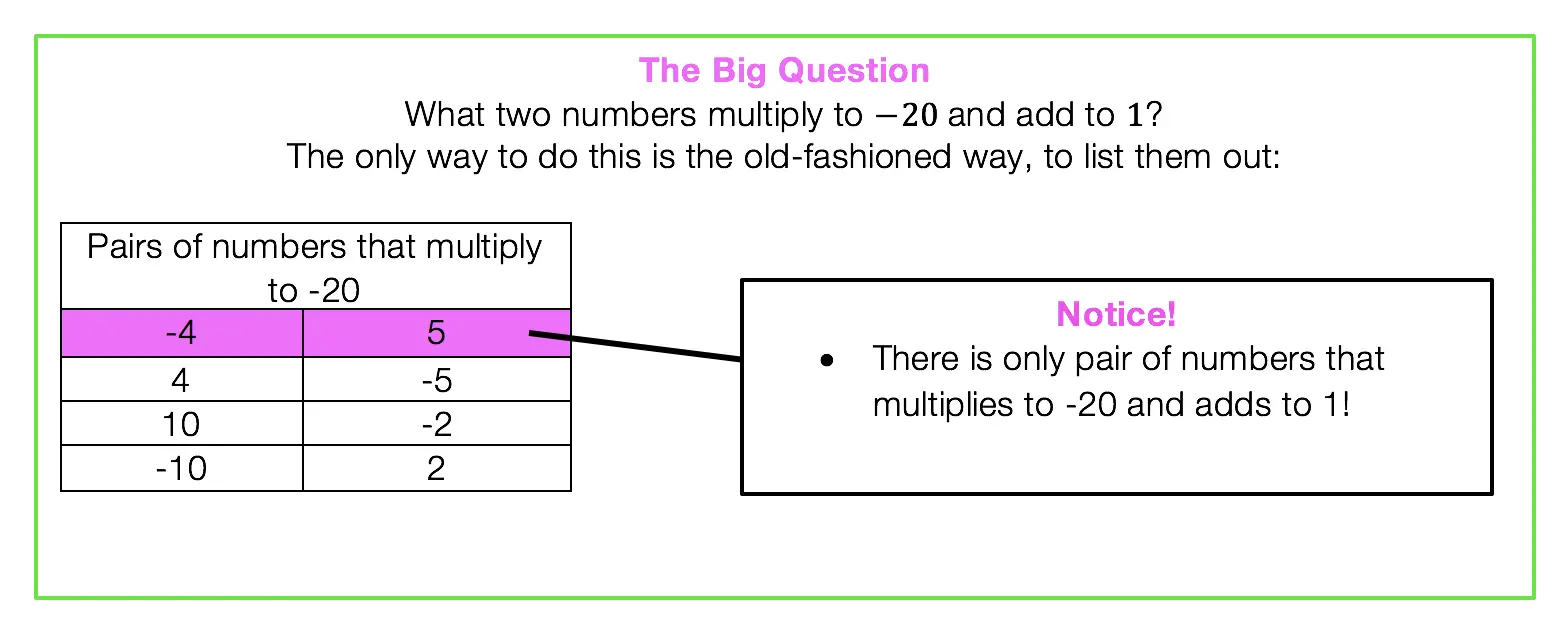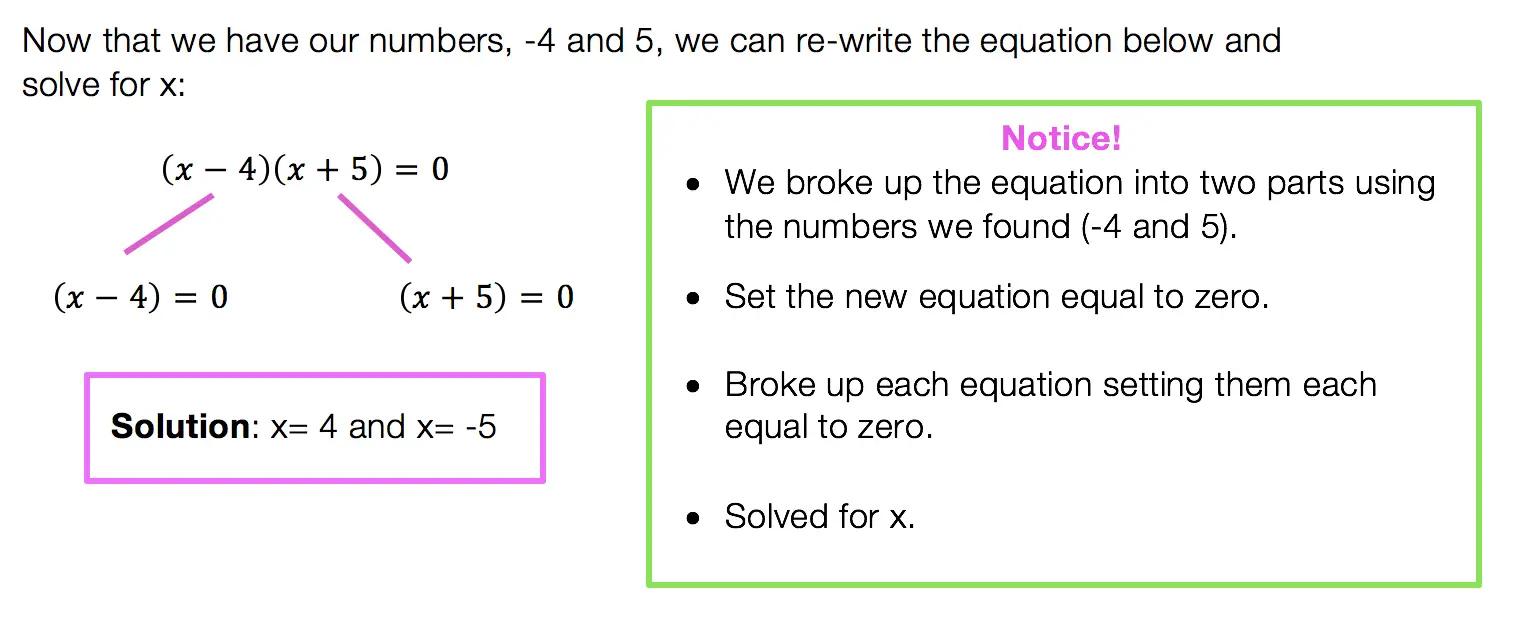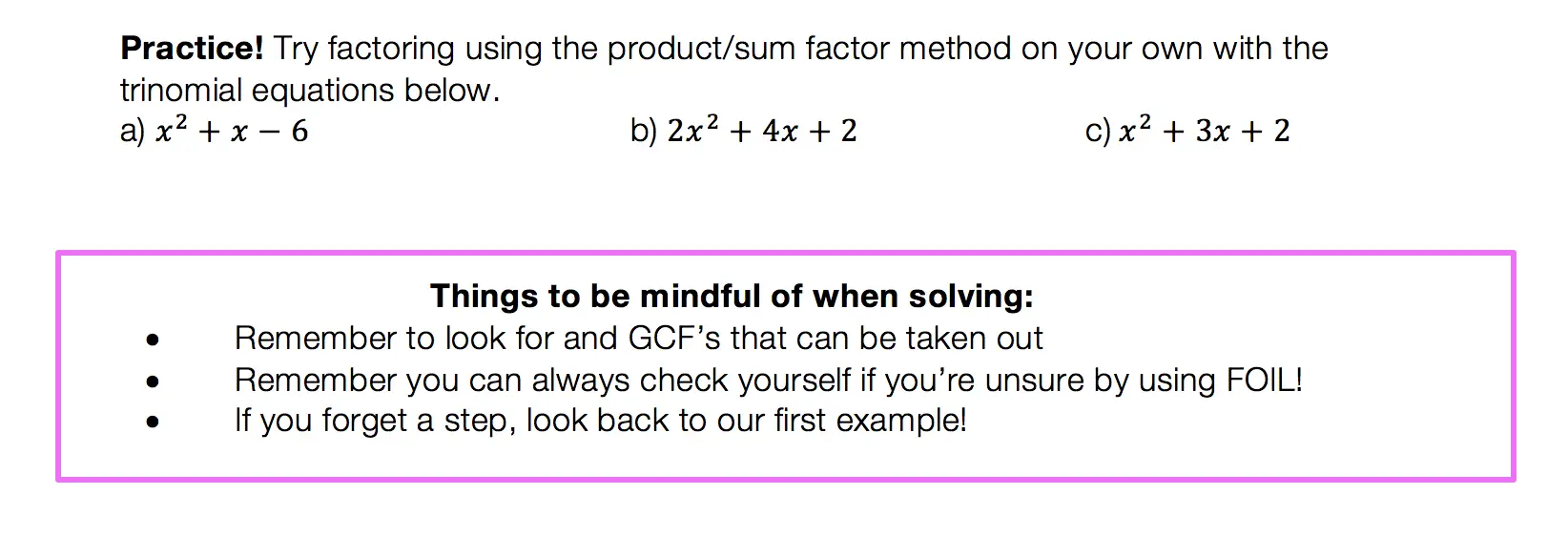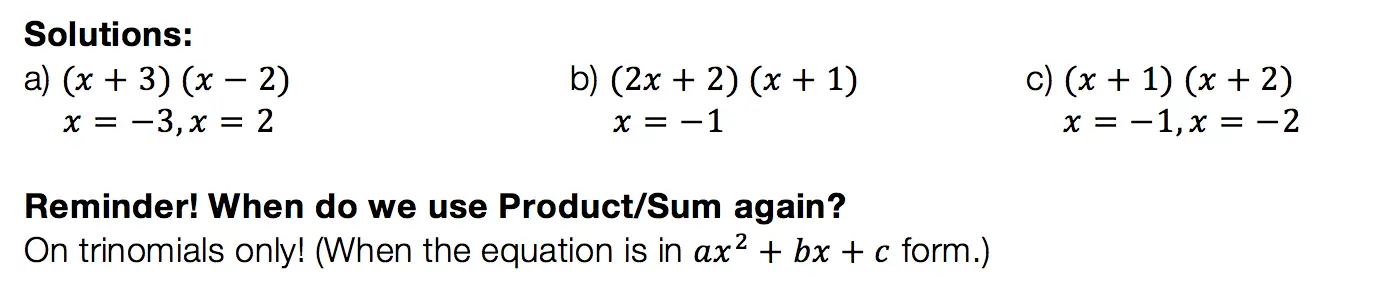## Difference of Two Squares DOTS)

Not to play favorites or anything, but DOTS is the easiest and most lovable of the factoring methods.  This factoring method just makes you feel all warm and fuzzy inside or maybe that’s just me).  Before we get into how to do DOTS, let’s talk about when?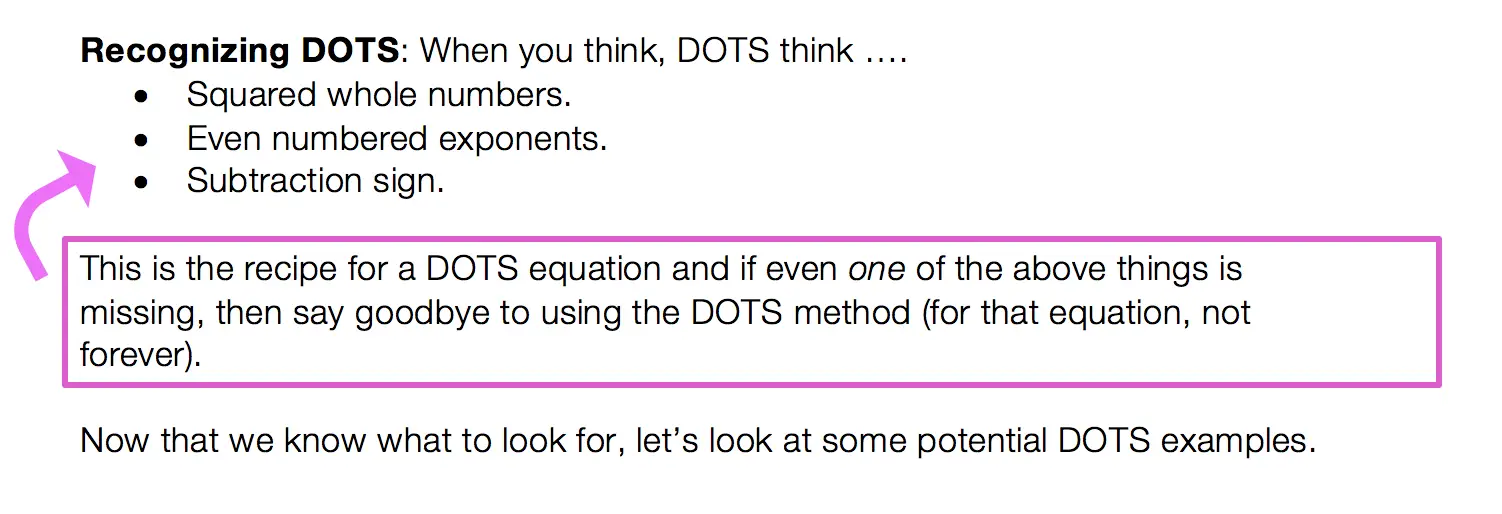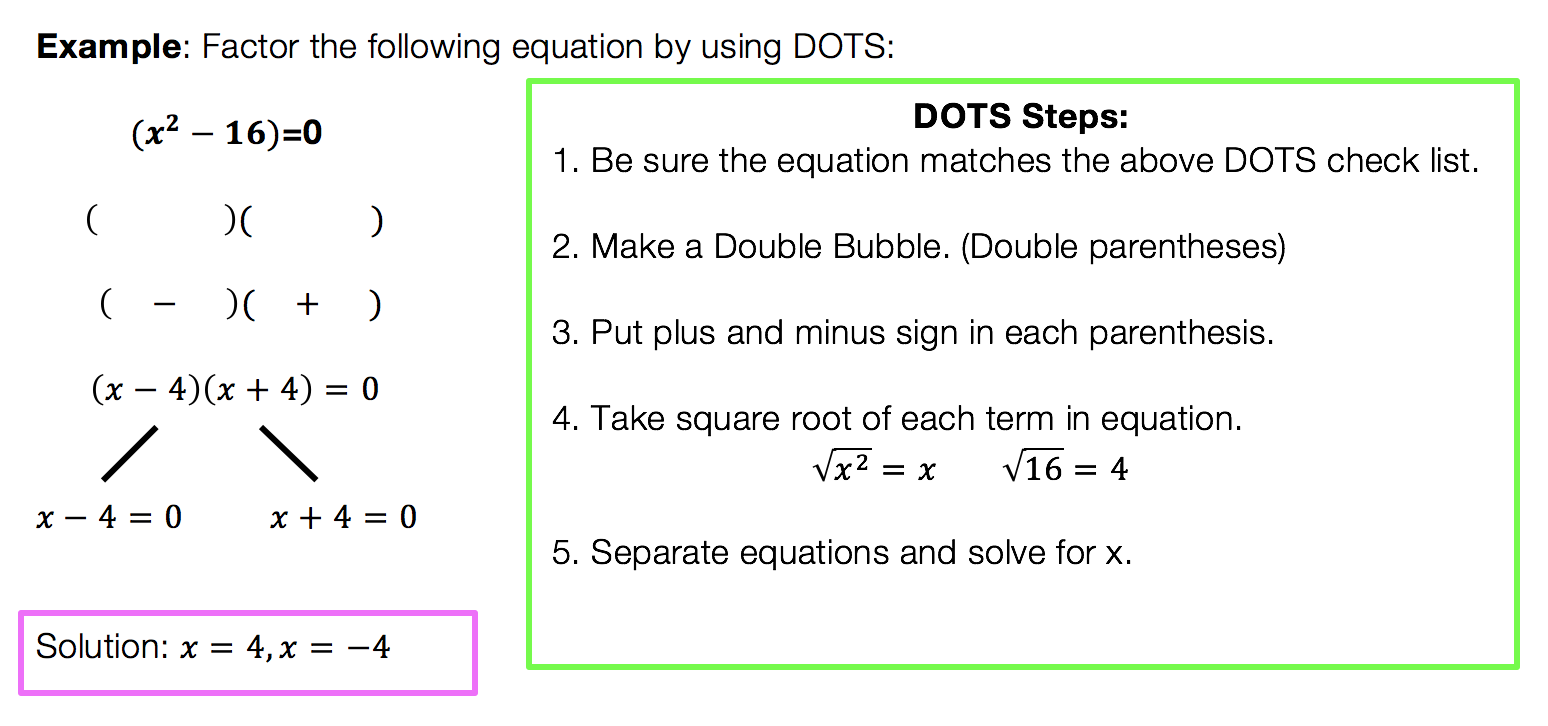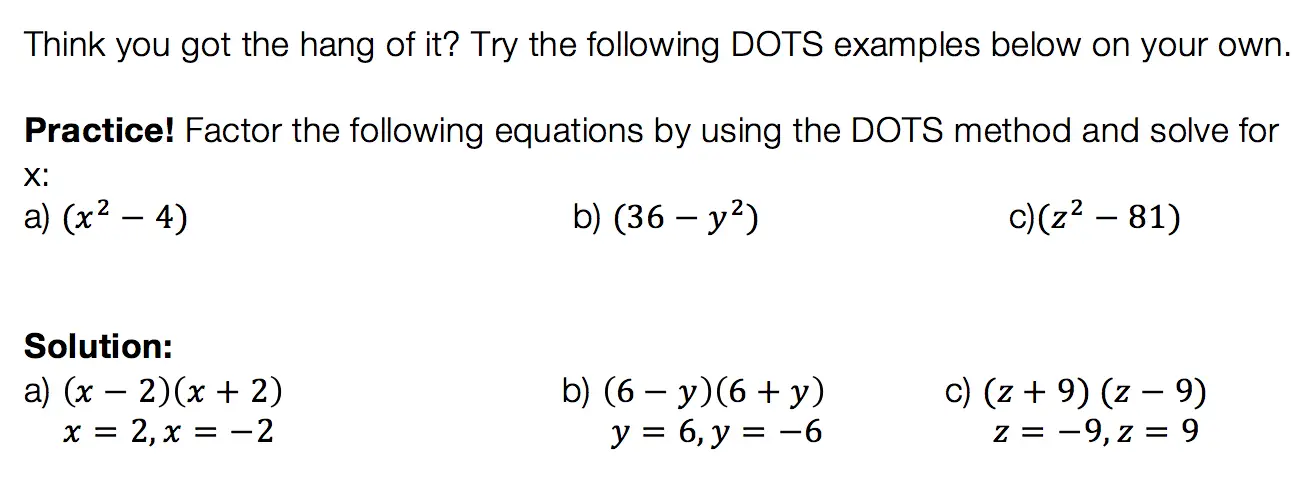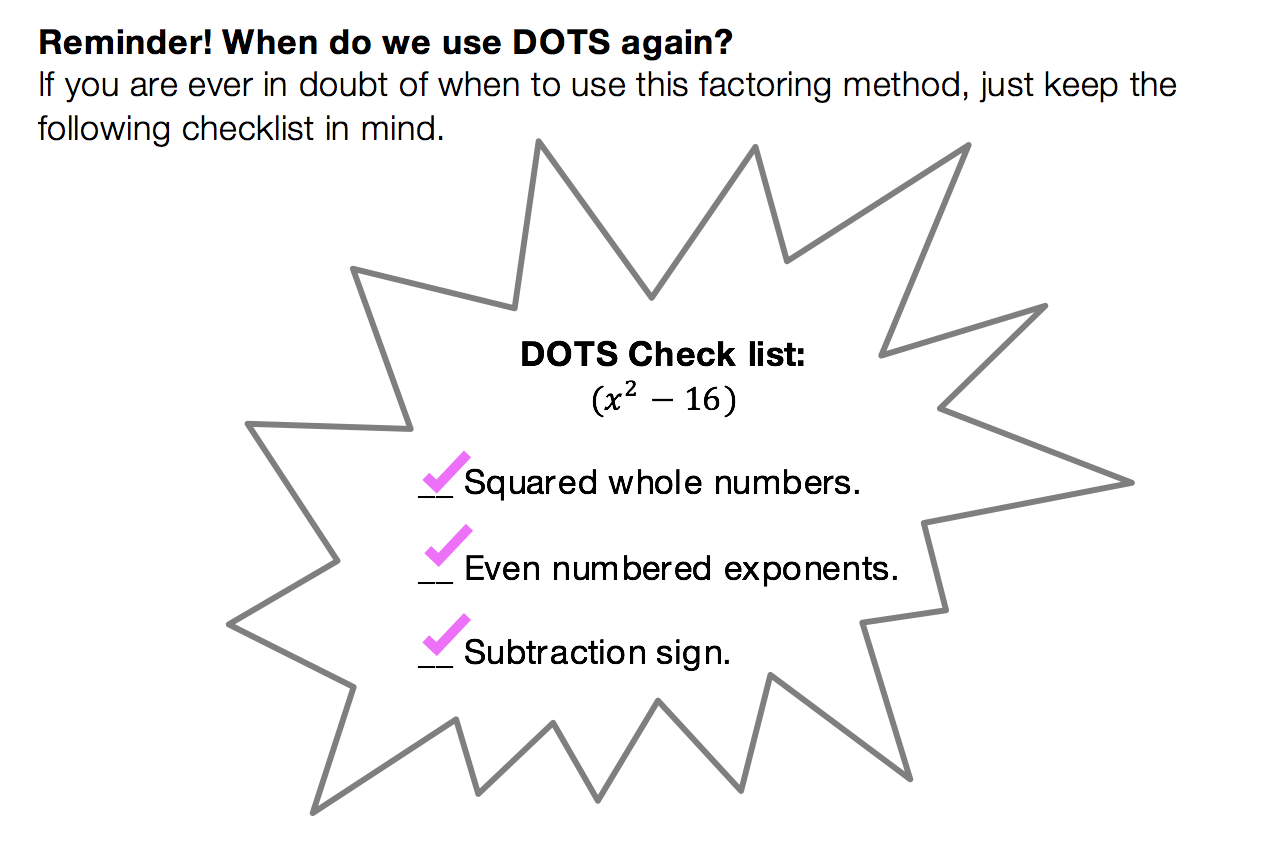We have heard of the quadratic equations, so how id the quadratic formula different?

The Answer: The Quadratic Formula is what we use to factor any trinomial. You can use product/sum on trinomials like we discussed earlier, but this may not always work out easy.  The Quadratic Formila on the other hand will work every time!

Low and behold, the Quadratic Formula: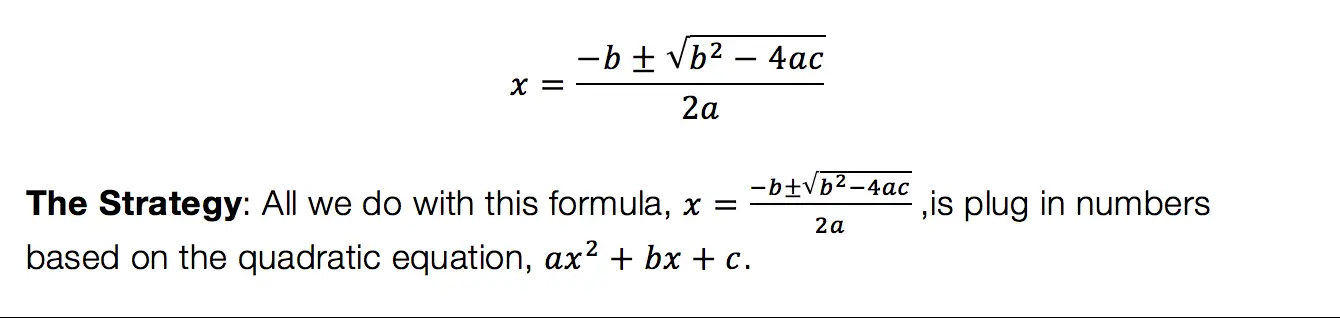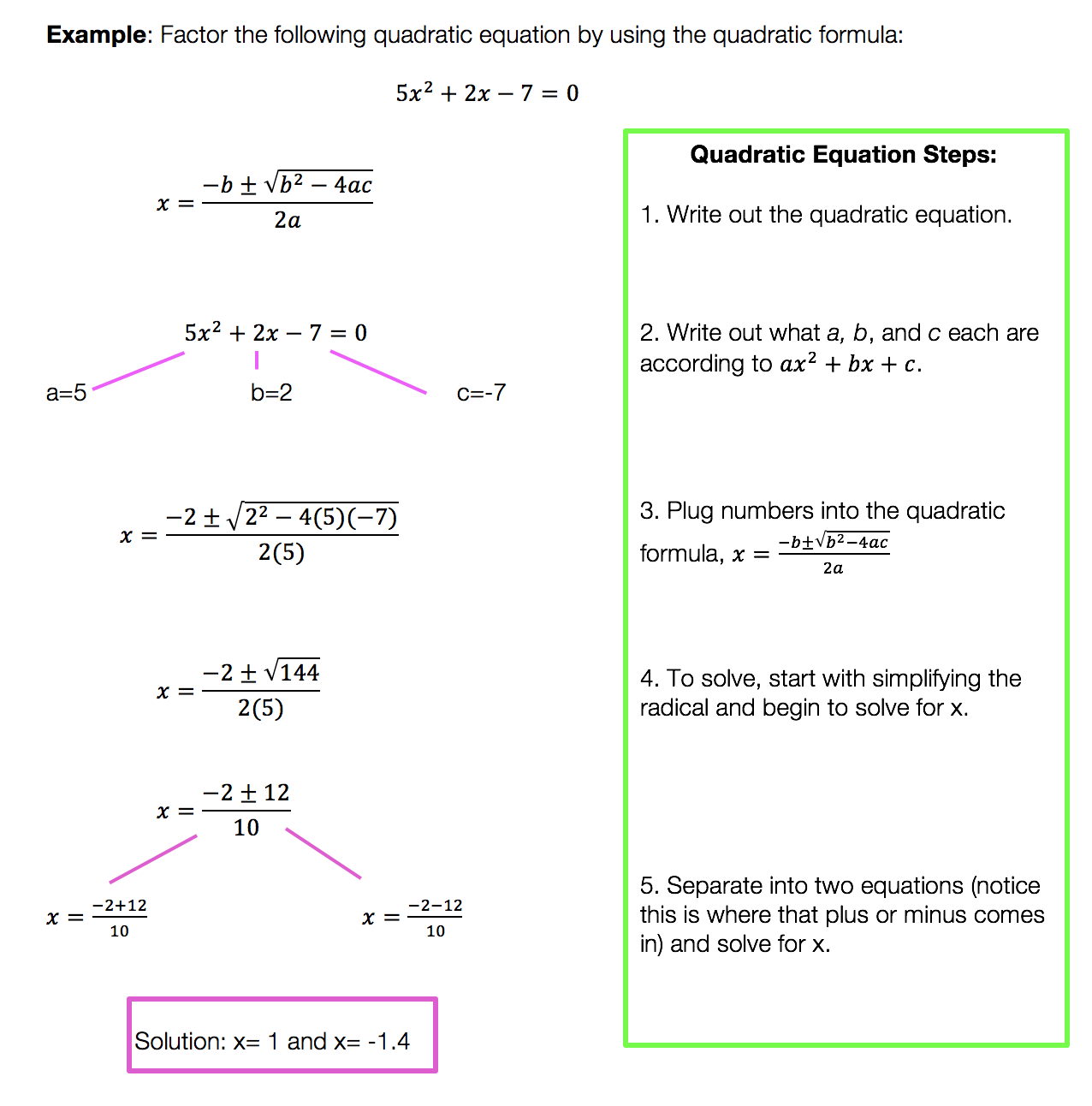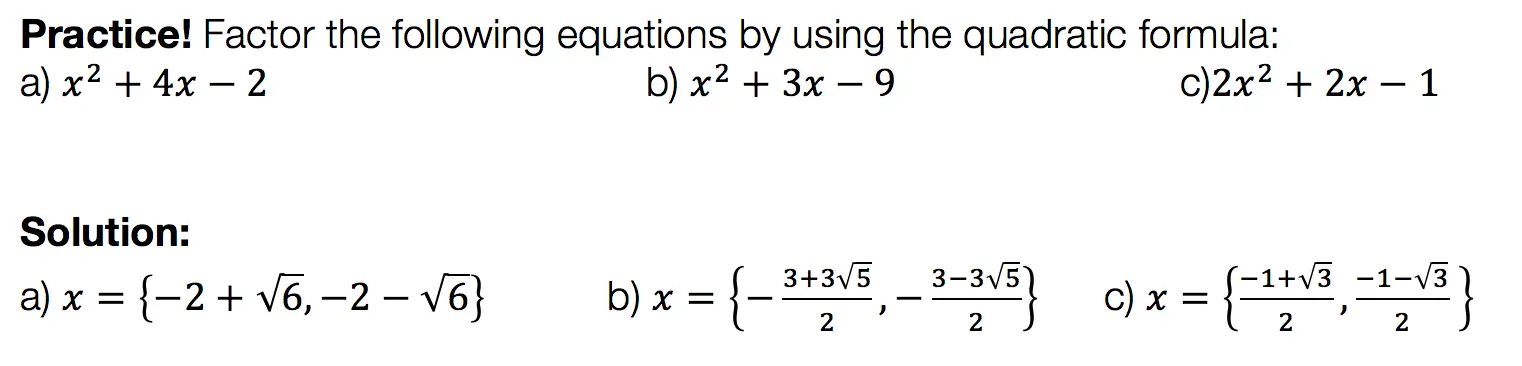Want more Mathsux?  Don’t forget to check out our Youtube channel and more below! And if you have any questions, please don’t hesitate to comment below. Happy Calculating! 🙂

Looking for more on Quadratic Equations and functions? Check out the following Related posts!

Factoring

Factor by Grouping

Completing the Square

The Discriminant

Is it a Function?

Quadratic Equations with 2 Imaginary Solutions

Imaginary and Complex Numbers

Focus and Directrix of a Parabola

## ‘Math Suks’ ~ Jimmy Buffett

Need to relax after studying? Close to wanting to “burn your textbook”? This super chill and somewhat cheesy song may help! Coming to you from Jimmy Buffett, the singer has a relaxed, island/ska like vibe to his music.  The lyrics come close to sounding like an after school special until the words “Math Sucks” are sung over and over again.  Enjoy! 🙂

‘Math Suks’ by Jimmy Buffet Lyrics:

If necessity is the mother of invention
Then I’d like to kill the guy who invented this
The numbers come together in some kind of a third dimension
A regular algebraic bliss.

Let’s start with something simple, like one and one ain’t three
Any two plus two will never get you five.
There are fractions in my subtraction and x don’t equal y
But my homework is bound to multiply.

Math suks math suks
I’d like to burn this textbook, I hate this stuff so much.
Math suks math suks
Sometimes I think that I don’t know that much
But math suks.

I got so bored with my homework, I turned on the TV.
The beauty contest winners were all smiling through their teeth.
Then they asked the new Miss America
Hey babe can you add up all those bucks?
She looked puzzled, then just said
“Math Suks”.

Math suks math suks
You don’t even have to spell it,
All you have to do is yell it…
Math suks math suks
Sometime times I think that I don’t know that much
But math suks.

Geometry, trigonometry and if that don’t tax your brain
There are numbers to big to be named
Numerical precision is a science with a mission
And I think it’s gonna drive me insane.

Parents fighting with their children, and the Congress can’t agree
Teachers and their students are all jousting constantly.
Management and labor keep rattling old sabers
Quacking like those Peabody ducks.

Math suks math suks
You don’t even have to spell it,
All you have to do is yell it…
Math suks math suks
Sometime times I think that I don’t know that much
But math suks

## Why Must We Complete the Square?: Algebra

Completing the Square: So many steps, such little time.  It sounds like it involves a square or maybe this is a geometry problem?  Why am I doing this again?  Why must we complete the square in the first place?

These are all the thoughts that cross our minds when first learning how to complete the square.  Well, I’m here to tell you there is a reason for all those steps and they aren’t that bad if you really break them down, let’s take a look!

Explanation:

I’m not going to lie to you here, there are a lot of seemingly meaningless steps to completing the square.  The truth is though (as shocking as it may be), is that they are not meaningless, they do form a pattern, and that there is a reason! Before we dive into why let’s look at how to solve this step by step:

Feeling accomplished yet!? Confused?  All normal feelings.  There are many steps to this process so go back and review, practice, and pay close attention to where things get fuzzy.

But the big question is why are we doing these steps in the first place?  Why does this work out, to begin with?

For those of you who are curious, continue to read below!

Want more Mathsux?  Don’t forget to check out our Youtube channel and more below! And if you have any questions, please don’t hesitate to comment below. Happy Calculating!

Need more of an explanation?  Check out why we complete the square in the first place here !

## Geometry: Congruent Triangles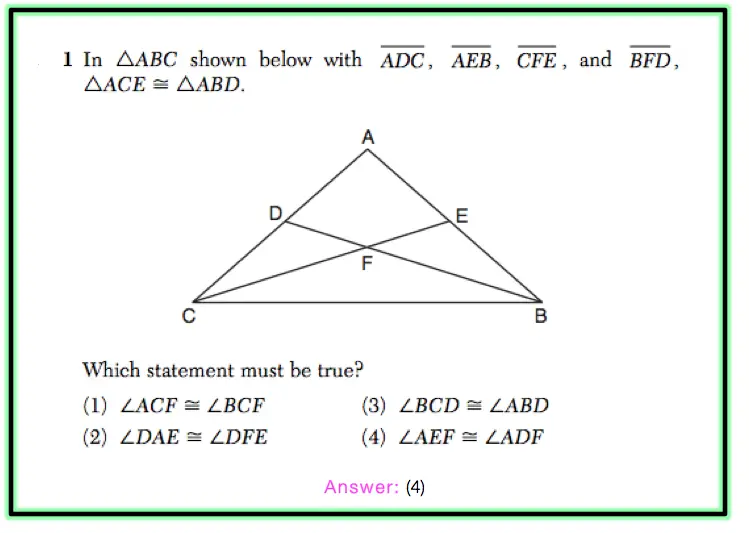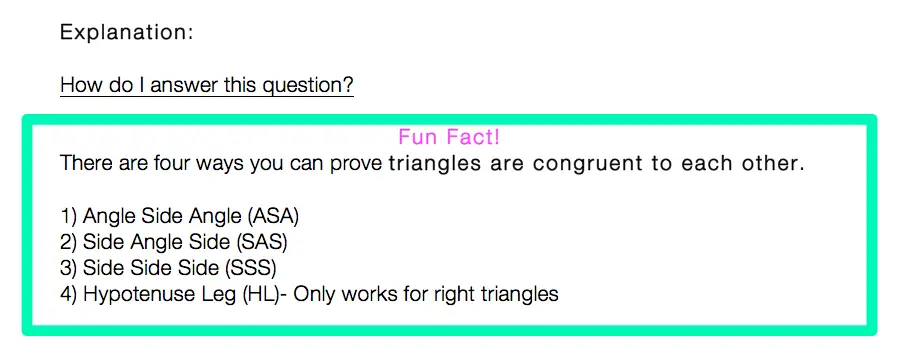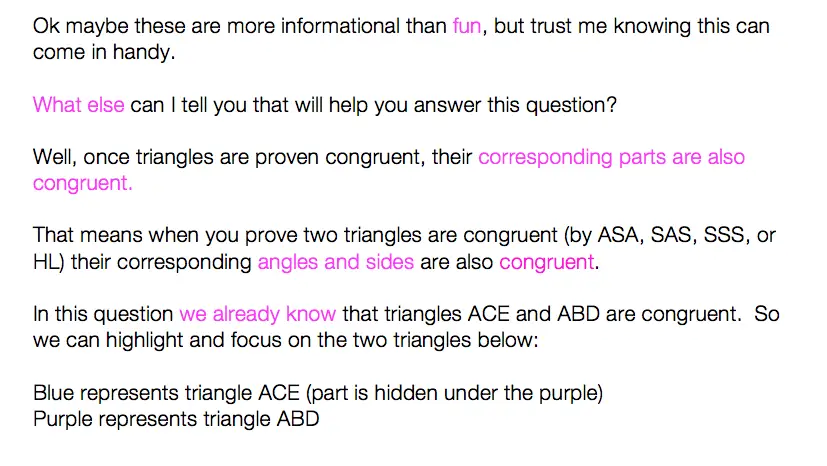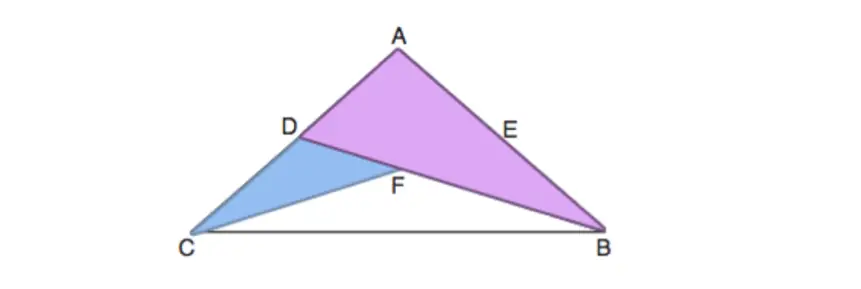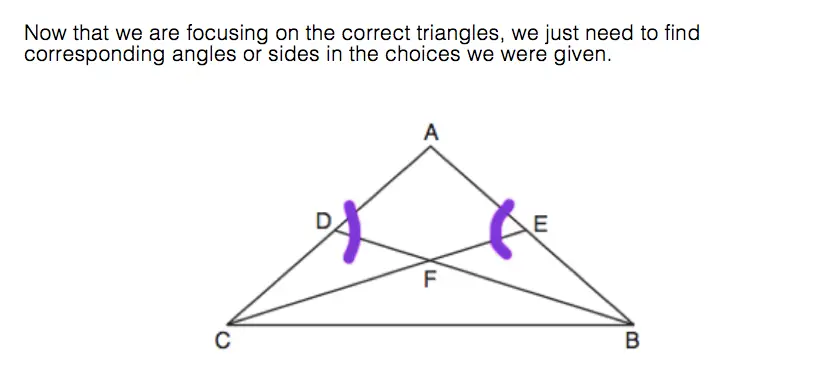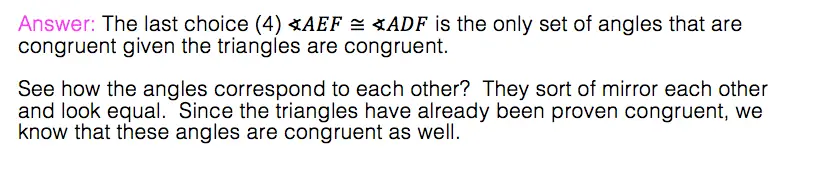Not bad, eh? If there is anything you have questions on please don’t hesitate to ask! 🙂 If you are looking to test out your skills on this topic even more, check out more challenging questions here.

And for more of me and my nerdy math jokes take a look at MathSux on

## How to Factor Trig Functions

Howdy math friends! In this post, we are going to learn how to factor trig functions algebraically.  This will combine our knowledge of algebra and trigonometry into one beautiful type of question! For more on trigonometric equations and the unit circle, check out this post here.

Solving trigonometric equations is very similar to solving the ho-hum everyday equations you solved all the way back in algebra 1, but this time we are solving for an angle value. If you need to refresh yourself on how to find x the old fashioned way via factoring be sure to check out this post here!

Solving trigonometric equations, sounds complicated? Well, you are correct, that does sound complicated. Is it complicated? Hopefully, you won’t find it that way after you’ve seen an example. We are going to find the value of x (value of the unknown angle) step by step in the following question:

## How do I answer this question?

The questions want us to solve for x, the unknown value of the angle.

Step 1: Our first step for solving trigonometric equations is to pretend this is any other algebraic equation and we want to solve for x (Note: this is the key to solving any trig equation). Thinking this way, allows us to move radical 2 to the other side, by adding it to both sides of the equation.

Step 2: Remember that secant is the inverse trigonometric function of cosine? Therefore we know that, secx is equal to 1/cosx and we can re-write the equation below, making the trigonometric function look a lot less complicated already!

Step 3: Now we need a value for x. This is where I turn to my handy dandy special triangles. There are two main special triangles in trignometry we can use to solve our equation, the 45 45 90 triangle and the 30 60 90 triangle. In this case, we are going to use the 45 45 90 special triangle, because its proportions include a value of radical 2, which is exactly what our equation has!

*Note- In this case we can use our special triangles, but in other examples, we may also need to use our knowledge of trig identities to re-write the original trigonometric function.

Below we can see the proportions that the 45 45 90 special triangle brings us. When we apply the basic rules of trigonometry and SOH CAH TOA, we see that we can find the value of our missing angle x, which is 45º.

*Another way we could have gotten 45º without using special triangles is by plugging in cos-1 (1/rad2) into our calculator, this would also give us the solution of 45º!

Step 4: We have a value for cos45º=1/rad 2, but remember we need its inverse and must flip the fraction, to find the value of our angle of the inverse of cosine, secx, which flipped, is going to be 45º.

***But wait! We are not done yet! We have found only one part to our solution, x=45º, which is great! But we need to ask ourselves, are there any other angle measures that could potentially give is the right solution as well!? And thats where we need to consult the unit circle!

Step 5: Using the unit circle below, we can identify what other values can work for our original trigonometric function. Based on the unit circle below, we can identify which trig functions (sin, cos, tan) are positive in which quadrants, positive and negative angles (positive counterclockwise, negative clockwise).

Notice that cosine is positive in Quadrants I and IV. That means there are two values that x can be (one in each quadrant). We already have x=45º from Quadrant i. In order to get the other value in Quadrant IV, we must subtract 360º-45º=315º using our reference angle (360º-θ) and giving us our other value for x, 315º, completing our answer!

The above example is similar to a linear equation, let’s look at another type of trigonometric equation that is more similar to quadratic equations.

## Factoring Trig Functions Example: Quadratic Equation

Step 1: First let’s move everything onto one side of the equation.  We can start by multiplying tanθ to both sides and distributing, multiplying (2tanθ-7)(tanθ). Then we can subtract the 4 from both sides.  Remember to solve trigonometric equations, we always want to treat them like a normal algebraic function.

Step 2: Now that we have something resembling a quadratic equation, we have something to work with! There are two main ways to solve for our unknown angle via factoring for this example, (1) The Quadratic Formula, or, (2) Factor by Grouping.  In this case, we are going to be using factor by grouping to find the value of our angle, and the solution to our problem, but the quadratic formula should work as well!

Factor by Grouping: First find the product, sum and factors of the equation.

Now that we have our factors, we can re-write our equation, converting -7tanθ into -8tanθ + tanθ.

Next, we split the equation down the middle and take the GCF of each side.

Finally, we can combine the values outside our parenthesis 2tanθ+1 and the matching values found within our parenthesis and tanθ-4.

Step 3: Now we can use our equation and solve for each angle value, by setting up each group found in the parenthesis equal to zero, just like we would when finding the value of x in a normal algebraic equation.

Based on finding our two angles for θ, -27º and 76º, we are able to find the angles that apply to our specific trigonometric equation listed below, based on where tanθ is positive and negative in each quadrant of the unit circle. In this case, the angle value of -27º, we look for the angle values where tangent is negative which are in quadrant ii and quadrant IV, and apply our reference angles. On the other hand, the angle value of 76º, is positive, therefore, we look for angles where tangent is positive, in quadrant i and quadrant iii, and apply our reference angles to get the correct value of each angle.

*Note! In the above two examples, we look at a cosine function and a tangent function, but the same exact methods apply to a sine function!

Think you are ready to solve trigonometric equations on your own? Check out the practice questions and solution to each below!

## Practice Questions:

Find the nearest degree to all values of θ or x in the interval 0º <  θ < 360º and 0º <  x < 360º:

When you are ready check each solution below!

## Solution:

Does the solution to each trig function make sense? Great! 🙂 Do you think it is clear as mud to solve trigonometric equations? Alas, I have failed. But I have not given up (and neither should you). Ask more questions, look for the spots where you got lost, do more research and never give up! 🙂

Hopefully, you enjoyed my short motivational speech. For more encouraging words and math, check out MathSux on the following websites! Sign up for FREE math videos, lessons, practice questions, and more. Thanks for stopping by and happy calculating! 🙂

## Related Trigonometry Posts:

The Unit Circle

Basic Right Triangle Trigonometric Ratios (SOH CAH TOA)

4545 90 Special Triangles

30 60 90 Special Triangles

Graphing Trigonometric Functions

Trig Identities

Transforming Trig Functions

Law of Cosines

Law of Sines

## PieceWise Functions NYS Regents: Algebra

Greetings math friends, students, and teachers I come in peace to review this piecewise functions NYS Regents question.  Are they pieces of functions? Yes. Are they wise?  Ah, yeah sure, why not? Let’s check out this piecewise functions NYS Regents question below and happy calculating! 🙂

1 value satisfies the equation because there is only one point on the graph where f(x) and g(x) meet.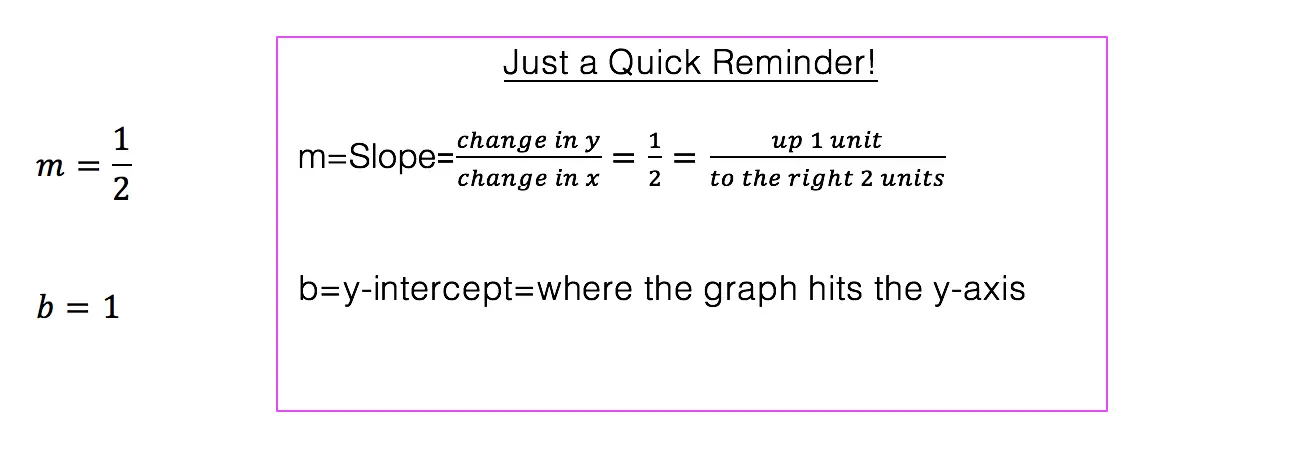*Note: For an in-depth review on how to graph an equation of a line step by step, check out this post here.

Does the above madness make sense to you? Great!

Need more of an explanation? Keep going! There is a way to understand the above mess.

How do I read this?

Looking at this piece-wise function: We want to graph the function 2x+1 but only when the x-values are less than or equal to negative 1.

We also want to graph 2-x^2 but only when x is greater than the negative one.

One way to organize graphing each piece of a piecewise function is by making a chart.

1. Lets start by making a chart for the first part of our function 2x+1:

Is it all coming back to you now?  Need more practice on piece-wise functions?Check out this link here and happy calculating! 🙂

Also, if you’re looking to nourish your mind or you know, procrastinate a bit check out and follow MathSux on these websites!

## Top 5 Favorite Math Websites

As we all love technology (I’m assuming since it has brought you here), I figured I should share my top favorite math sites.  These are great resources for learning things you don’t understand, practicing for an upcoming test or if you’re like me, it’s great for re-learning/teaching purposes.  Yes, even I forget how to do things and these websites are a great help.  I know the anticipation is getting to you so I’ll just get to the good stuff now. Here are my top 5 favorite math websites:

1. Khan Academy : This is a non profit over in Silicon Valley.  They have a great range of subjects (k-college level)  and explain everything via easy to follow videos.
2. Regent’s Prep: The title is pretty self explanatory, but this helps with reviewing for the New York State Regent’s.  This site has a lot of great examples and answers.
3.  MathSux:  So fabulous, no explanation is required. 😀
4.  Knewton:  Pretty cool things are happening at this web address.  You can make an account, answer some questions and the questions will adjust according to your answers figuring out what you need help in.
5. Kahoot: Fast and easy quizzes that are good for an entire classroom or for studying alone.  Either way there are lots of bright and happy colors that make you forget you’re studying anything in the first place.

So those are the goods, the contraband, the info you can’t find anywhere else (all lies).  Hope you find these websites useful. Let me know if you end up using any of them.  🙂

## Pre-Calc: The Factor Theorem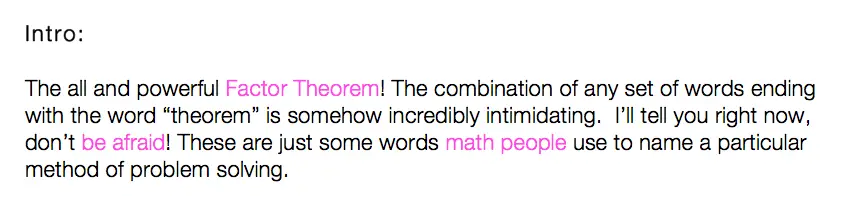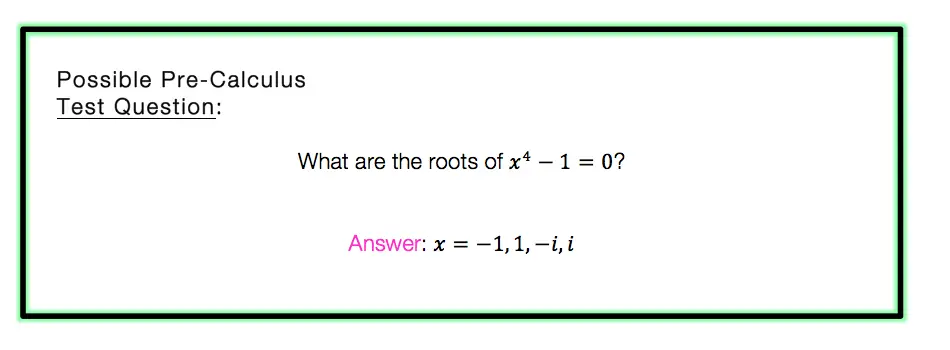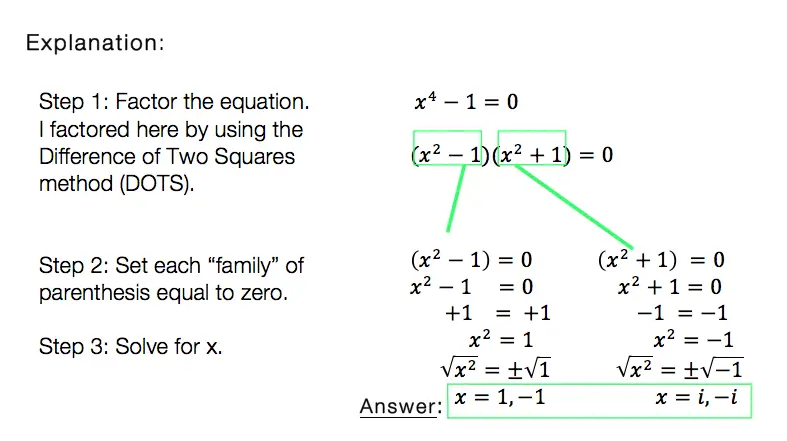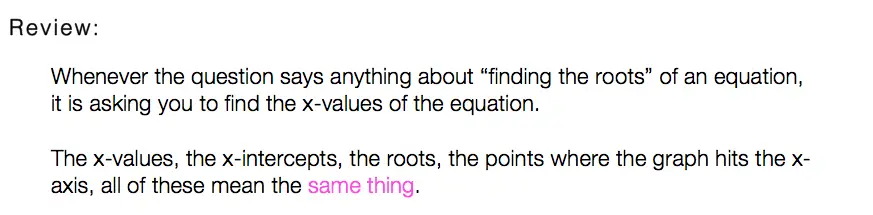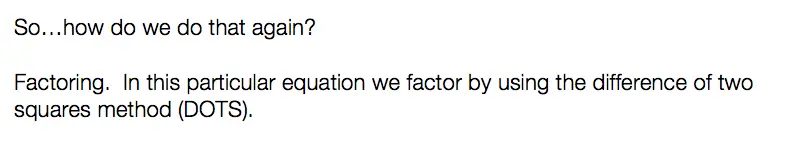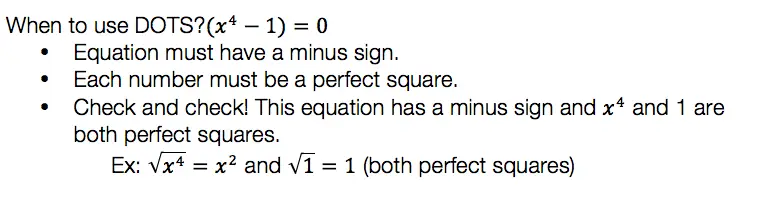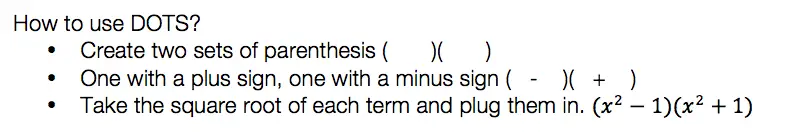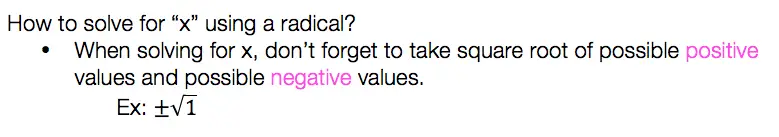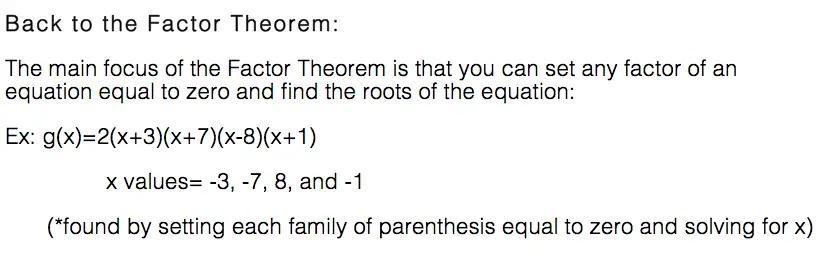So what do we think of the factor theorem?  Not as intimidating as you thought, you probably have been using it for years without realizing it.

For more math fun check me out on Twitter and Facebook.  Until next time, keep it real and happy mathing! 🙂

For related posts on factoring methods that apply the factor theorem, check the following lessons out below:

How to Factor Quadratic Equations

Why must we complete the square?

4 Ways to Factor a Trinomial

Factor By Grouping

## Factor by Grouping: Algebra 2/Trig.

Hey math friends! In this post, we are going to go over Factor by Grouping, one of the many methods for factoring a quadratic equation.  There are so many methods to factor quadratic equations, but this is a great choice, for when a is greater than 1. Also, if you need to review different types of factoring methods, just check out this link here.  Stay curious and happy calculating! 🙂 Before we go any further, let’s just take a quick look at what a quadratic equation is: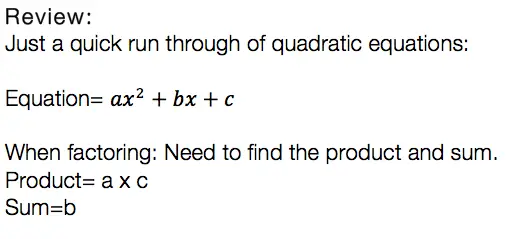Usually, we can just find the products, the sum, re-write the equation, solve for x, and be on our merry way.  But if you notice, there is something special about the question below. The coefficient “a” is greater than 1/. This is where factor by grouping comes in handy!Now that we know why and when we need to factor by grouping lets take a look at our Example: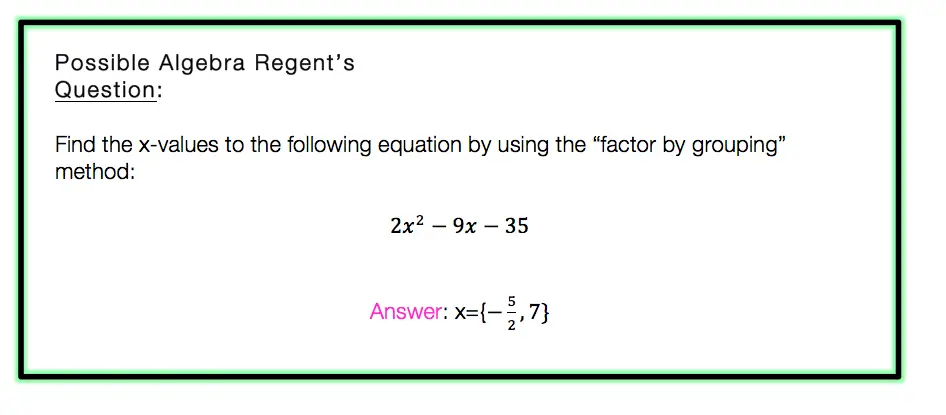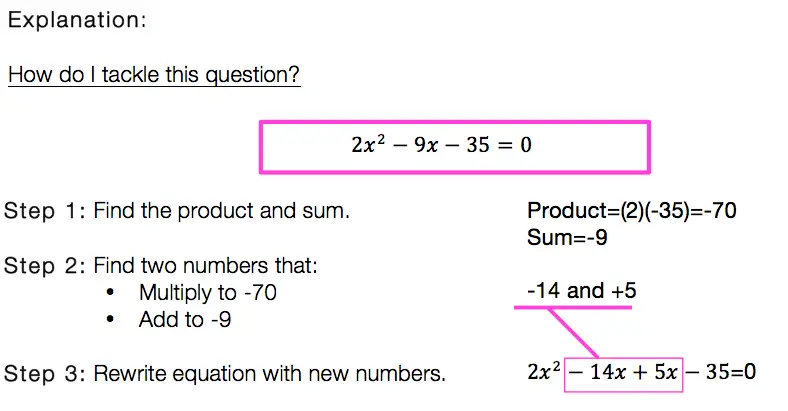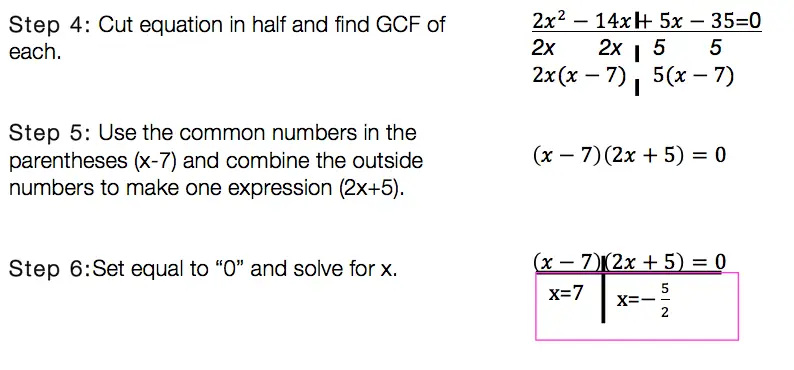Factor By Grouping: Hard to solve? No.  Hard to remember? It can be, just remember to practice, practice practice! Also, if you are in need of a review of other methods of factoring quadratic equations, click this link here.

Want more Mathsux?  Don’t forget to check out our Youtube channel and more below! And if you have any questions, please don’t hesitate to comment. Happy Calculating! 🙂

Looking for more on Quadratic Equations and Functions? Check out the following Related posts!

Factoring Review

What is the Discriminant?

Completing the Square

4 Ways to Factor a Trinomial

Is it a Function?

Imaginary and Complex Numbers

Quadratic Equations with 2 Imaginary Solutions

Focus and Directrix of a Parabola

## Equation of A Circle: Geometry

Equation of A Circle

Ahoy math friends and welcome to Math Sux! In this post, we are going to learn about the equation of a circle, mainly how to write it when given a circle on a coordinate plane. We’ll see how to find the radius and center from the graphed circle and then see how to transfer that into our equation of a circle. And we’re going to do all of this step by step with the following Regents question. Stay curious and happy calculating! 🙂

## How do I Answer this Question?

Let’s mark up this coordinate plane and take as much information away from it as possible. When looking at this coordinate plane, we can find the value of the circle’s center and the circle’s radius.

Now that found the center and radius, we can fit each into our equation:

Center=(-3,-4)